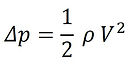###### Workshop #9 - Introduction to Jets

An air jet is a good means for providing convective heat transfer with a surface. This is why you blow on your food if it is too hot. A sketch of a typical an industrial jet is shown in the figure. Large arrays of hot air jets are often used in drying processes. Fans pressurize large plenums with many small orifice holes in a plate that then impinge on the opposing surface.

A common correlation for the heat transfer on the flat surface in the impingement region beneath an impinging jet is

where Re = V*d/v and Nu = h*d/k

The thermal conductivity is k and the kinematic viscosity is v. The effective diameter of the jet at the exit is d and the velocity is V. The velocity can be determined from Bernoulli’s equation. in terms of the plenum pressure p relative to atmospheric.

where ρ is the fluid density. Therefore, the pressure difference is

Your challenge today is to measure the convective heat transfer coefficient when you blow on something. Tape the heat flux sensor to your piece of aluminum and place it on a surface at room temperature. Put the wire connection side down next to the metal to get a positive heat flux. Tape the second thermocouple so that the bead is sitting above the sensor, but not touching it. This will provide a measure of the air temperature from your lungs. Start the data acquisition and blow as long and hard as you can. Then stop and save your data.

Note that this is a highly transient event and the direction of heat flux and temperatures may switch momentarily, giving what appears to be negative values of h. Use the plots of heat flux and temperature to interpret your heat transfer coefficients.

1. Give the maximum value of heat flux that you achieved.

Maximum qʺ =

2. Record the temperatures when the maximum heat flux occurs.

Ts =

Tair =

3. Calculate the corresponding heat transfer coefficient and Nusselt number. Look in a mirror to estimate the diameter of your mouth when you blow. Use this as the characteristic length, Lc = d =

h =

Nu = h*d/k =

4. From the Nusselt number correlation given in the Introduction, determine the corresponding velocity that this heat transfer measurement estimates, V =

5. Your lungs can provide an air pressure of several inches of water. What lung pressure is required to provide the air velocity you calculated in 4? Assume that Bernoulli equation applies. (249 Pa = 1 in. H2O)

Δp =                                                          Is this value reasonable?

6. Show equations and calculations.
Air properties at 300 K
Thermal conductivity, k =

Density, ρ =

Kinematic viscosity, v =